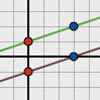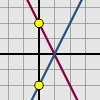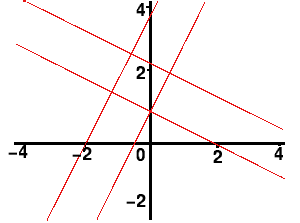#### You may also like### Parallel Lines

How does the position of the line affect the equation of the line? What can you say about the equations of parallel lines?### Diamond Collector

Collect as many diamonds as you can by drawing three straight lines.### Reflecting Lines

Investigate what happens to the equations of different lines when you reflect them in one of the axes. Try to predict what will happen. Explain your findings.

# Enclosing Squares

##### Age 11 to 14Challenge Level

Here's a problem to work at with your graphic calculator or graph-plotting package on a computer.

If you plot the following lines

 $\begin{eqnarray} y &=& 2x + 1\\ y &=& 2x + 4 \\ y &=& -0.5x + 1\\ y &=& -0.5x + 2.5 \end{eqnarray}$the lines will enclose a square.

Can you find other sets of sloping lines that enclose a square?

If you are given the equations of two parallel lines

$y = ax + b$ and $y = ax + c$

can you explain how to find the equations of the other two lines that would enclose a square, if you know that one of the vertices is at $(0,b)$?

[In the example at the top, $a = 2$, $b = 1$ and $c = 4$]